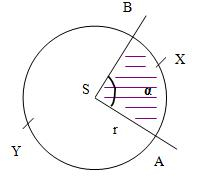# Convex angle

There is a circle k (S; r) and a point A, which lies on this circle. There is also a point B on the circumference, for which it is true that in one direction it is five times further from the point A than in the opposite direction (around the circumference of the circle). Find the magnitude of the BSA convex angle.

BSA =  60 °

### Step-by-step explanation:Did you find an error or inaccuracy? Feel free to write us. Thank you!

#### Units of physical quantities:

We encourage you to watch this tutorial video on this math problem: